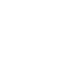# Jan 19 ACT & SAT Question of the Day

http://sat.collegeboard.org/practice/sat-question-of-the-day?src=R&questionId=20130119 (This link takes you to today’s question. If you use my archive, you will see the question related to my SAT explanation for that date. However in my first few blogs, I didn’t use a date in my link and you won’t get the proper question. Sorry.)

The answer is B.  Read the SAT staff’s explanation.  It is one of two ways to do this question.  But there is a much easier way to do it!  I hope you’ve learned and memorized the “vertex formula.”  If not, you should do so.  I’ve been looking at SAT questions on released tests for over 20 years and don’t remember seeing this kind of question until the last few weeks and I’ve seen it twice on the Question of the Day.  Forewarned is forearmed; so, I’m guessing they are now going to be putting it on the test.  On both occasions they have had this concept on the Question of the Day, the SAT explanation was the hard way to do the question.

Just memorize the vertex formula.  The vertex of a quadratic equation (the highest or lowest point of the parabolic graph) is located at some point (x,y) of a coordinate graph.  The x value will be calculated as x=-b/2a.  The y value will be calculated as y=c-(b2/a).  In this question, a=1, b==-6, and c=8 just like if you were using the quadratic formula.

For the line y=a, you know that y is a horizontal line because the y value of the line is constant.  So, it could always be at 3 no matter what the x value is.  It could be at -2, or 10.  All you know at this point is that it is at a.  The question tells you the quadratic function (or equation) is only intersected at one point.  Since quadratic functions are parabolas, the line y=a, which touches the parabola at only one point, must touch it only at the vertex.  (If it intersected the parabola anywhere else it would have to intersect it twice since a parabola is a “V” with a rounded bottom or an upside-down “V.”)  That means it has to touch it at the very bottom or top.  In this case, the “V” is shaped this way, i.e., it opens upwards, since the value of a is positive.  (If the equation has a negative coefficient, the “V” opens downward.)

So you simply need to use the vertex formula for y.  Plugging in the values of a, b, and c, we get 8 – (36/4) = 8-9 = -1.  I think that’s a lot easier than the SAT staff’s explanation.  Their explanation works; it just takes a lot longer to do it their way.  I think that they forget the SAT is timed!  They are constantly giving explanations that take much more time.

As usual, doing the math isn’t hard.  Keep in mind this is the “Reasoning Test.”  Figuring out the horizontal line must touch at the vertex since it only intersected the parabola in one place was the reasoning part.  Using the vertex formula just took a few seconds.  Doing the math that the SAT staff used to answer this question took much longer.  Oh well, that’s why you read my explanations anyway.  Did you think they were going to give away the secrets to improving your score?  Now you know better.

I wonder what the ACT folks are up to this morning.

http://www.act.org/qotd/ (The ACT staff does not put a date on their questions so if you click on an archived blog, you’ll get today’s question and the old explanation. Sorry. The SAT staff has dated their questions; so, the archive is helpful. The ACT folks simply don’t do that.)

The answer is F.  This is a simple detail question which is clearly answered in the passage.  (You will rarely, if ever, see a detail question on the SAT.)  The challenge is to find the answer.  The topic sentences for each paragraph are your guides.  Use them.  Since the question is about “trial by ordeal,” scan the topic sentences and you see that the paragraph that starts at line 36 is about “ordeals.”  Focus on that paragraph and you find lines 51-56 answer the question.

What is critical about doing ACT and SAT practice questions isn’t being able to answer this or any other specific question.  You have to use each one to learn and practice a specific strategy.  Then apply those strategies on test day.  The Wizard’s strategy you should learn from this question is that the topic sentences provide you the organization of the passage and can be used (especially on the ACT) to go find answers.

Osceola students–if you’ve done the practice test and have a question about any of the items, let me know and I’ll help you.  The test is only a week away.

If you are taking the January SAT and/or February ACT, time is running short. I recommend you watch my online Tips and Tricks videos to help you prepare. In addition to the free ones on the home page, it only costs \$3 to watch an hour of my best test-taking techniques for taking the SAT and ACT tests.

The Wizard